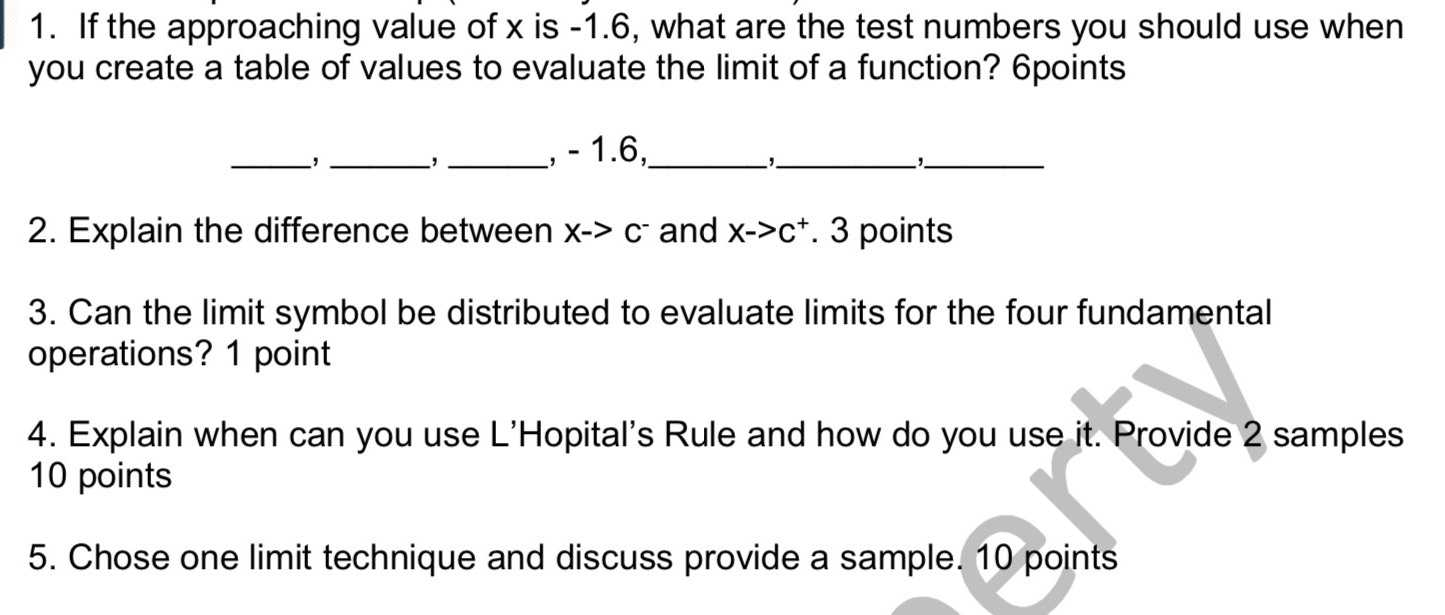### ¿Todavía tienes preguntas de matemáticas?

Pregunte a nuestros tutores expertos
Algebra
Pregunta1. If the approaching value of $$x$$ is $$- 1.6$$ , what are the test numbers you should use when you create a table of values to evaluate the limit of a function?

2. Explain the difference between $$x - > c ^ { - }$$ and $$x - c ^ { + }$$

3. Can the limit symbol be distributed to evaluate limits for the four fundamental operations?

4. Explain when can you use L'Hopital's Rule and how do you use it. Provide $$2$$ samples

5. Chose one limit technique and discuss provide a sample.